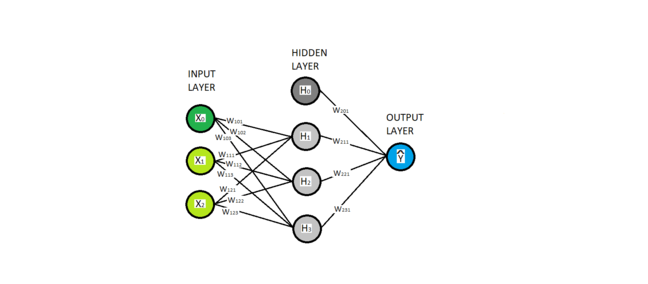## Want to keep learning?

This content is taken from the The Open University & Persontyle's online course, Advanced Machine Learning. Join the course to learn more.
2.17

# Basics

Neural networks are a popular and powerful class of supervised learning models used for both regression and classification. In this course we present an overview of the most basic type of neural network, the multi-layered perceptron. There are other types, often customized with particular uses in mind, such as convolutional neural networks which have performed exceptionally well in image analysis, or recurrent networks, which have performed very well on problems involving sequential data such as dynamic system modeling and natural language analysis.

Let’s begin by introducing the graphical symbolism we will use with neural networks. In this, we will represent variables by nodes. We will wish to distinguish between input features, latent variables and the model’s estimate of the target variable. These are colored green, gray and blue respectively, and are given in layers of the same type known as input, hidden and output layers. You will notice that X0 and H0 are shaded differently from other nodes in their layer. These are dummy or bias variables, which always take the value 1.Latent variables are not new - we have already encountered them in our discussion of polynomial regression when we looked at feature transformations in the first module. Latent variables are functions of the inputs. In the network diagram this relationship is given by edges. Interpreting edges as directed from left to right, each variable/node with edges coming into it is a function of the variables/nodes from which the edges come, and the weights associated with these edges. You can see that each variable in the hidden and output layers is a function of all variables in the previous layer, except for the bias variable H0 which always takes the value 1. The weights have three indices standing for layer, from node and to node, such that wabc is the weight associated with the edges from node b in layer a to node c in layer a+1 (understanding layers as ordered from left to right). It is the weights that form the parameters of a neural network model and which are fitted to data during training.

#### Aside: Deep Learning & Deep Neural Networks

It is common to distinguish between deep and shallow neural networks based on the number of hidden layers they possess, with deep networks having more (and sometimes very many) hidden layers. It is common to refer as deep to any network that has more than one hidden layer, though sometimes this adjective is used comparatively to discuss networks that have more or fewer layers. Deep learning involves the use of deep neural networks.

The distinction is important, in that some of the non-linear transformations that were commonly used in shallow networks lead to poor results in deeper networks.

The functions used in neural networks fall into two categories. For hidden nodes, the functions used are known as activation functions. We look at a number of common activation functions below, and we see that they are normally a non-linear function of the weighted sum of the variables in the previous layer. The weights of this weighted sum being, of course, the edge weights. Producing a layer of hidden nodes via such functions amounts to a non-linear feature transformation. Deep neural networks will have multiple hidden layers, and are therefore performing a series of feature transformations on the original input features.

In most neural networks, the functions used to estimate the target variable given the final hidden layer are very familiar: In the case of regression, linear regression is used. In the case of classification, logistic regression is used (when working with non-binary target variables, multinomial logistic regression is used, but it is referred to as softmax in the neural network literature). In both cases, the weights on edges into the target variable form the coefficients of the linear/logistic regression model. Accordingly, you should see a neural network as a series of one or more non-linear features transformations followed by linear/logistic regression. You can think of the feature transformations as seeking to find the coordinate system where linear/logistic regression can be most effectively applied.

However, there is an important difference between the feature transformations occuring in a neural network and those occuring in simpler statistical models such as polynomial regression. In the latter case, we (the data scientist) specify before hand the transformations to use. In the neural network case, although we specify the form of the transformations, the actual transformations to use are learnt from an examination of the data as part of the training process. This is because the transformations are parameterized by the weights, and the values of the weights are fitted during training.

### Adaptive and optimal basis models

Non-linear feature transformations are common in both simple and advanced statistical learning techniques. As noted, simple techniques specify in advance some intuitively plausible set of feature transformations to use, such as the power series used in polynomial regression. Advanced machine learning techniques that make use of feature transformations tend to fall into two groups: (i) those that use feature transformations whose parameters are trained from the data, such as (and principally) neural networks, and which are known as adaptive basis models; and (ii) those that use types of feature transformations that are proven to be optimal (in some mathematically defined sense). We will see examples of this second type when we examine kernel methods.